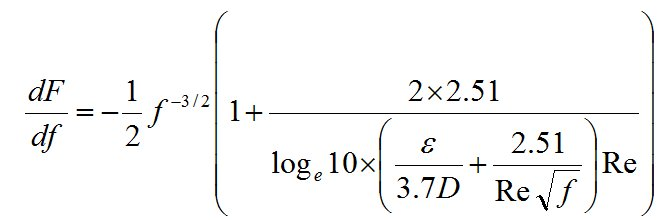Friction - small vs large pipes

Jacques Chaurette

May 2016

download in pdf formatIt is an interesting fact that friction is higher in small pipes than larger pipes for the same velocity of flow and the same length. Friction is determined by the Darcy-Weisbach equation which in turn relies on the friction parameter given from the Colebrook equation.Figure 1 Darcy-Weisbach equation for calulating the friction factor.Figure 2 Colebrook equation for calulting the friction parameter.

In the latter equation the relative roughness ε/D is a major component of friction. This number is large for small tubes which makes friction higher.

The following graph shows the variation in friction factor for typical pump discharge velocities between 8-13 ft/s and pipes ranging in size from 0.5 to 12 inches. For these calculations I have used the absolute roughness for steel (0.00015 ft) which is a very common material. Small tubes could be in polyethylene or some clear soft plastic material both of which would have a similar roughness or lower than steel.Figure 3 The friction factor vs velocity for different velocities.

As we can see, the friction factor is low for large pipes and high for small ones. It is as much as 50 times higher for the velocity range and pipe sizes considered.

Thankfully, since most projects that use small tubes have generally short distances to cover, the higher friction does not become a problem. The other factor is that most small projects use pumps that produce relatively low head that will generate low velocities therefore lower friction.

What about power? If we compare the friction power or power required to overcome friction we find that the larger pipes will require more power because of the higher flow rates as expected.Figure 4 The friction power vs velocity for different velocities.

Calculations

The friction parameter was determined by using the Moody diagram (shown below). It is possible to calculate the friction parameter directly by the Colebrook equation by using the Newton-Raphson iteration technique which is in my book and I have included here for convenience. The rest of the calculations are straight forward and here is an example:

Velocity is given

The flow rate for power calculation is:The relative roughness is:The friction factor from Darcy-Weisbach:Friction:Friction power:The Newton-Raphson Iteration Technique

Since the value for f in the Colebrook equation cannot be explicitly extracted from the equation, a numerical method is required to find the solution. Like all numerical methods, we first assume a value for f, and then, in successive calculations, bring the original assumption closer to the true value. Depending on the technique used, this can be a long or slow process. The Newton-Raphson method has the advantage of converging very rapidly to a precise solution. Normally only two or three iterations are required.

The Colebrook equation is:The technique can be summarized as follows:

1. Re-write the Colebrook equation as:2. Take the derivative of the function F with respect to f:3. Give a trial value to f. The function F will have a residue (a non-zero value). This residue (RES) will tend towards zero very rapidly if we use the derivative of F in the calculation of the residue.For n = 0 assume a value for f0, calculate RES and then f1, repeat the process until RES is sufficiently small (for example RES < 1 x l0-6).

The Newton-Raphson iteration technique is a method that converges very rapidly to a solution. You need to provide a seed value for fn-1 to start the iteration and an acceptable error RES which you can make very small.

You can find an example of the solution at this link:

www.pumpfundamentals.com/solving_colebrook.htm

which makes use of an Excel spreadsheet and the Goal/Seek function.